Signal Generator

Generate various waveforms

• Library:

•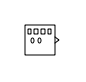Description

Supported Operations

The Signal Generator block can produce one of four different waveforms:

• sine

• square

• sawtooth

• random

You can express signal parameters in hertz or radians per second. Using default parameter values, you get one of the following waveforms:

WaveformScope Output

Sine wave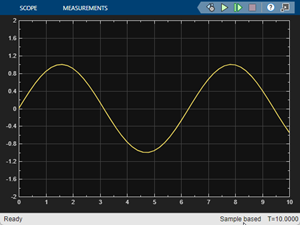Square wave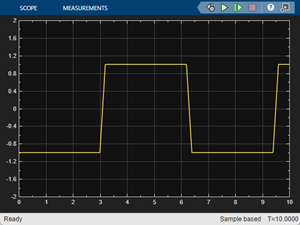Sawtooth wave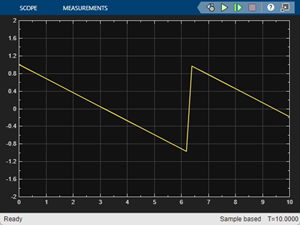Random wave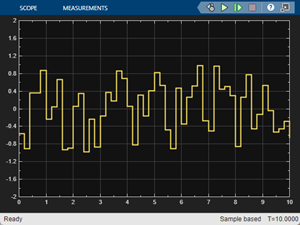A negative Amplitude parameter value causes a 180-degree phase shift. You can generate a phase-shifted wave at other than 180 degrees in many ways. For example, you can connect a Clock block signal to a MATLAB Function block and write the equation for the specific wave.

You can vary the output settings of the Signal Generator block while a simulation is in progress to determine quickly the response of a system to different types of inputs.

The Amplitude and Frequency parameters determine the amplitude and frequency of the output signal. The parameters must be of the same dimensions after scalar expansion. If you clear the Interpret vector parameters as 1-D check box, the block outputs a signal of the same dimensions as the Amplitude and Frequency parameters (after scalar expansion). If you select the Interpret vector parameters as 1-D check box, the block outputs a vector (1-D) signal if the Amplitude and Frequency parameters are row or column vectors, that is, single-row or column 2-D arrays. Otherwise, the block outputs a signal of the same dimensions as the parameters.

Solver Considerations

If your model uses a fixed-step solver, Simulink® uses the same step size for the entire simulation. In this case, the Signal Generator block output provides a uniformly sampled representation of the ideal waveform.

If your model uses a variable-step solver, Simulink might use different step sizes during the simulation. In this case, the Signal Generator block output does not always provide a uniformly sampled representation of the ideal waveform. To ensure that the block output is a uniformly sampled representation, add a Hit Crossing block directly downstream of the Signal Generator block. These models show the difference in Signal Generator block output with and without the Hit Crossing block.

Model That Uses a Variable-Step SolverSignal Generator Block Output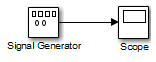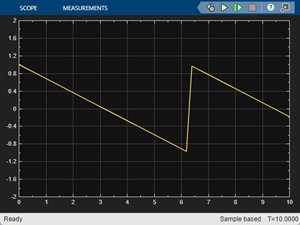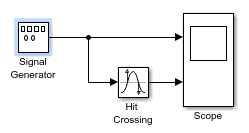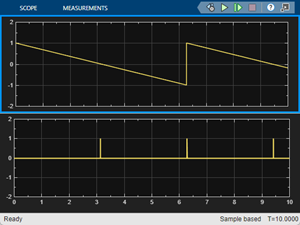Ports

Output

expand all

Output signal specified as one of these waveforms.

• sine

• square

• sawtooth

• random

Data Types: double

Parameters

expand all

Specify the wave form.

Programmatic Use

 Block Parameter: WaveForm Type: character vector Values: 'sine' | 'square' | 'sawtooth' | 'random' Default: 'sine'

Specify whether to use simulation time or an external signal as the source of values for the waveform time variable. If you specify an external source, the block displays an input port for connecting the source.

Programmatic Use

 Block Parameter: TimeSource Type: character vector Values: 'Use simulation time' | 'Use external signal' Default: 'Use simulation time'

Specify the amplitude of the generated waveform.

Programmatic Use

 Block Parameter: Amplitude Type: character vector Values: real scalar Default: '1'

Specify the frequency of the generated waveform.

Programmatic Use

 Block Parameter: Frequency Type: character vector Values: real scalar Default: '1'

Specify the signal units as Hertz or rad/sec.

Programmatic Use

 Block Parameter: Units Type: character vector Values: 'rad/sec' | 'Hertz' Default: 'rad/sec'

Select this check box to output a vector of length N if the Constant value parameter evaluates to an N-element row or column vector.

• When you select this check box, the block outputs a vector of length N if the Constant value parameter evaluates to an N-element row or column vector. For example, the block outputs a matrix of dimension 1-by-N or N-by-1.

• When you clear this check box, the block does not output a vector of length N if the Constant value parameter evaluates to an N-element row or column vector.

Programmatic Use

 Block Parameter: VectorParams1D Type: character vector Values: 'on' | 'off' Default: 'on'

Block Characteristics

 Data Types double Direct Feedthrough yes Multidimensional Signals yes Variable-Size Signals no Zero-Crossing Detection no